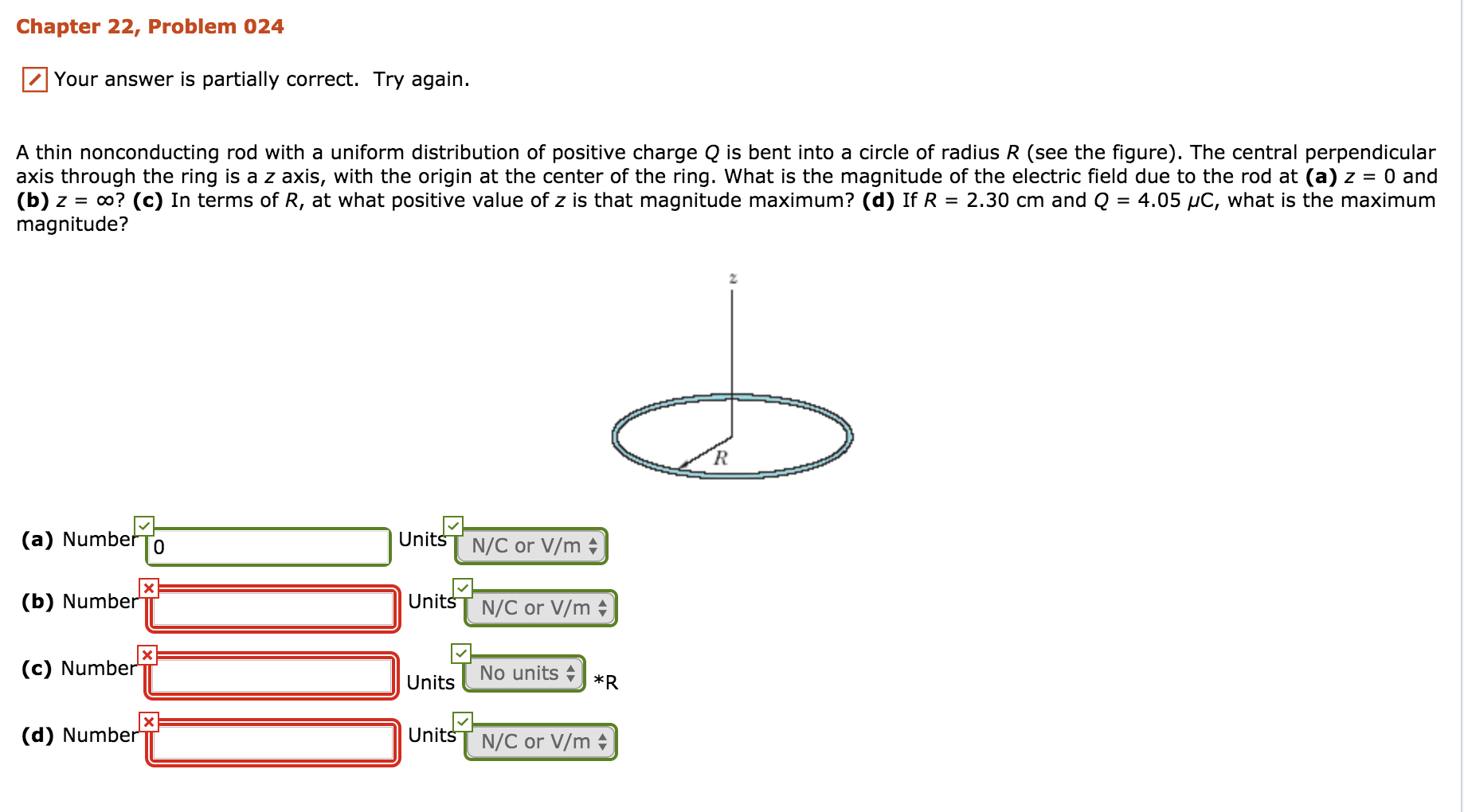Chapter 22, Problem 024Your answer is partially correct. Try again.A thin nonconducting rod with a uniform distribution of positive charge Q is bent into a circle of radius R (see the figure). The central perpendicularaxis through the ring is a z axis, with the origin at the center of the ring. what is the magnitude of the electric field due to the rod at (a)2-0 and(b)2 = oo? (c) In terms of R, at what positive value ofz is that magnitude maximum? (d) If R = 2.30 cm and Q = 4.05 pC, what is the maximummagnitude?(a) NumberToUnits N/C or V/mUnitsT N/C or V/mUnits No units 1Units N/C or V/m(b) Numbe(c) Number(d) Number

Questionhelp_outlineImage TranscriptioncloseChapter 22, Problem 024 Your answer is partially correct. Try again. A thin nonconducting rod with a uniform distribution of positive charge Q is bent into a circle of radius R (see the figure). The central perpendicular axis through the ring is a z axis, with the origin at the center of the ring. what is the magnitude of the electric field due to the rod at (a)2-0 and (b)2 = oo? (c) In terms of R, at what positive value ofz is that magnitude maximum? (d) If R = 2.30 cm and Q = 4.05 pC, what is the maximum magnitude? (a) NumberTo Units N/C or V/m UnitsT N/C or V/m Units No units 1 Units N/C or V/m (b) Numbe (c) Number (d) Number fullscreen
Step 1

Hello, since there are multiple subparts posted, we will answer the first three subparts.

(a)The equation for the magnitude of electric field due to a circular ring of radius R along the perpendicular axis from the center at a distance z is given by

Step 2

(c)The derivative of the magnitude electric field with respect to z will be zero at the value of z where the magnitude is maximum. The condition to find the value of z where the magnitude will be maximum is given by

Step 3

It is asked to find the po...

Want to see the full answer?

See Solution

Want to see this answer and more?

Our solutions are written by experts, many with advanced degrees, and available 24/7

See Solution
Tagged in

Electric Charges and Fields